# Commutative property facts for kids

Kids Encyclopedia Facts

The commutative property says that the order of the numbers when adding or multiplying can be changed without changing the answer. For example, both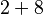$2+8$ and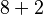$8+2$ are equal to 10, and both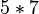$5*7$ and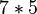$7*5$ are equal to 35. This can be done with any numbers, or with more than two numbers.

The definition of commutative property of addition is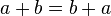$a+b=b+a$. a and b are variables and can be any number.

Some operations like dividing are not commutative. For instance,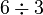$6\div3$ is 2, but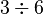$3\div6$ is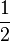$\frac{1}{2}$. Subtraction is not commutative either: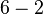$6-2$ is 4, but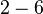$2-6$ is negative 4.

In higher mathematics like calculus, there are other commutative operations besides adding and multiplying.

## Images for kidsIn Spanish: Conmutatividad para niños